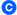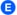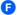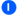# FC-100V

Close

• The colors may differ slightly from the original.

AAA × 1 (R03)

## Features• Plastic keys
• Comes with new slide-on hard case
• Power supply:One AAA-size battery
• Approximate battery life:17,000 hours continuous display of flashing cursor
• Dimensions:13.7(H) × 80(W) × 161(D) mm
• Approximate weight:110g

### Direct mode key

A bank of mode keys provides you with one-touch access to the mode you need.1.Simple interest
Interest amount, principal and interest

2.Compound interest
Payment period, interest rate, deposit amount, future value

3.Investment Appraisal (cash flow)
Net present value method, internal rate of return method, payback period method, etc.

4.Amortization
Monthly payment, principal and interest to date

5.General and function
Virtually the same functions as a standard calculator.

6.Statistical and regression
Statistical calculations using input sample data.

7.Interest rate conversion
Nominal interest rate and effective interest rate conversion

8.Cost, selling price,or margin
Calculation of any of the above values after inputting the other two

9.Day or date calculations
Virtually the same as a standard calculator, with some variation in the input methodFC-200V FC-100V
Simple interestCompound interestInvestment Appraisal (cash flow)AmortizationInterest rate conversionCost,selling price,or marginDay or date calculationsDepreciationBond calculationBreak-even pointGeneral and FunctionStatistical and regression### Easy operation with parameters

A full-dot 4-line display provides easier scrolling between parameters and simplifies input, confirmation, and editing.### Calculate the result.The result appears immediately after you press the SOLVE key.

### Create shortcuts.Once you use a parameter value or setting in a calculation, you can assign it to a shortcut key for instant recall whenever you need it. This feature is great for repeat calculations.

## Specifications

• Non Programmable

•AAA × 1 (R03)

•10 + 2 digits 10-digit mantissa + 2-digit exponential display.

•Dot matrix display High-resolution screen provides beautiful looking graphs every time.

•Plastic keys Designed and engineered for easy operation.

• Non Graphing

• AAA-size Battery

• Basic Statistics Standard statistics functions such as Mean, SUM, Standard Deviation, and Regression

• Basic Mathematical Functions Trigonometric, Exponential logarithmic, etc.

• Financial Financial functions are built in, making financial calculations easy.